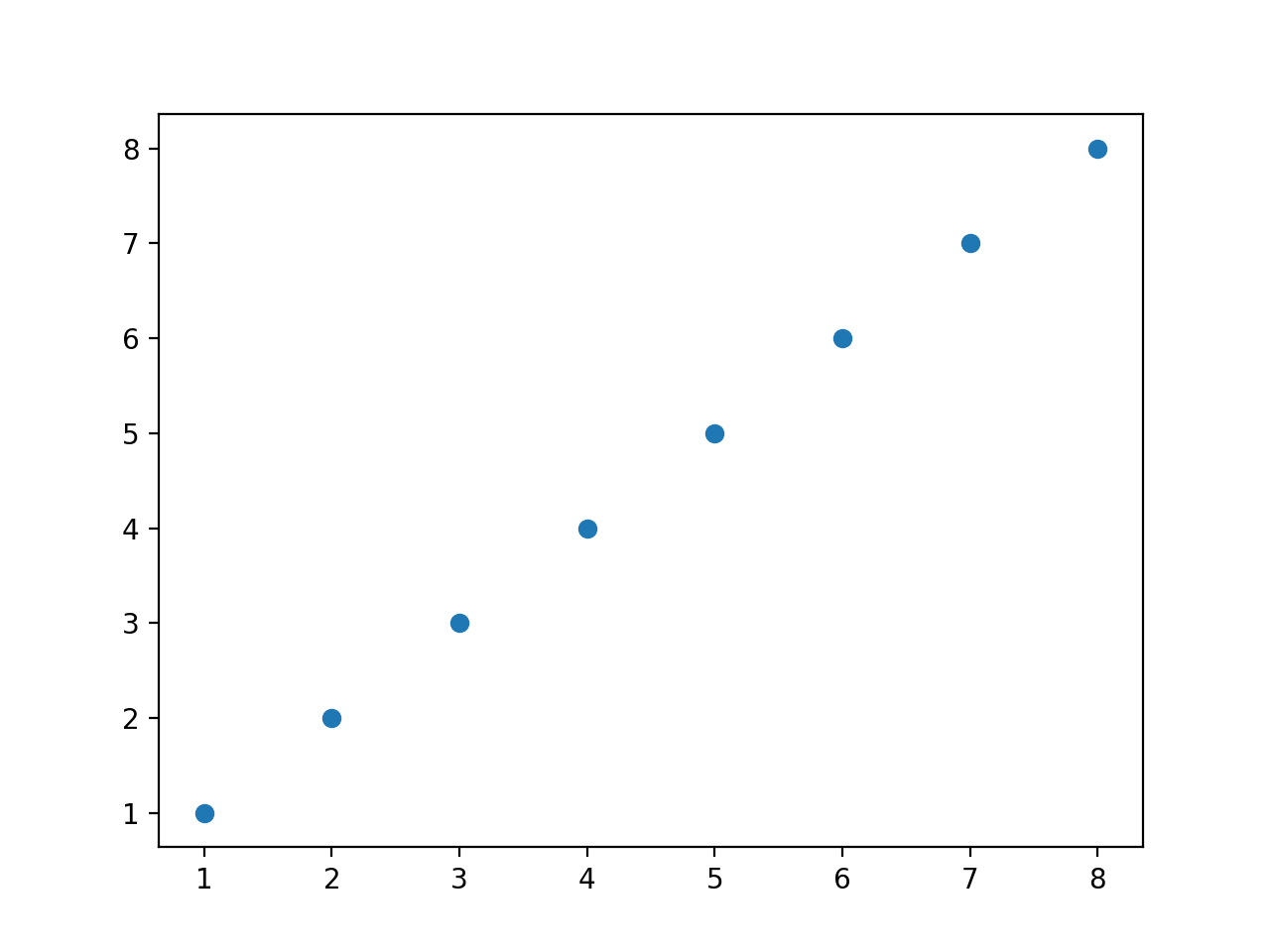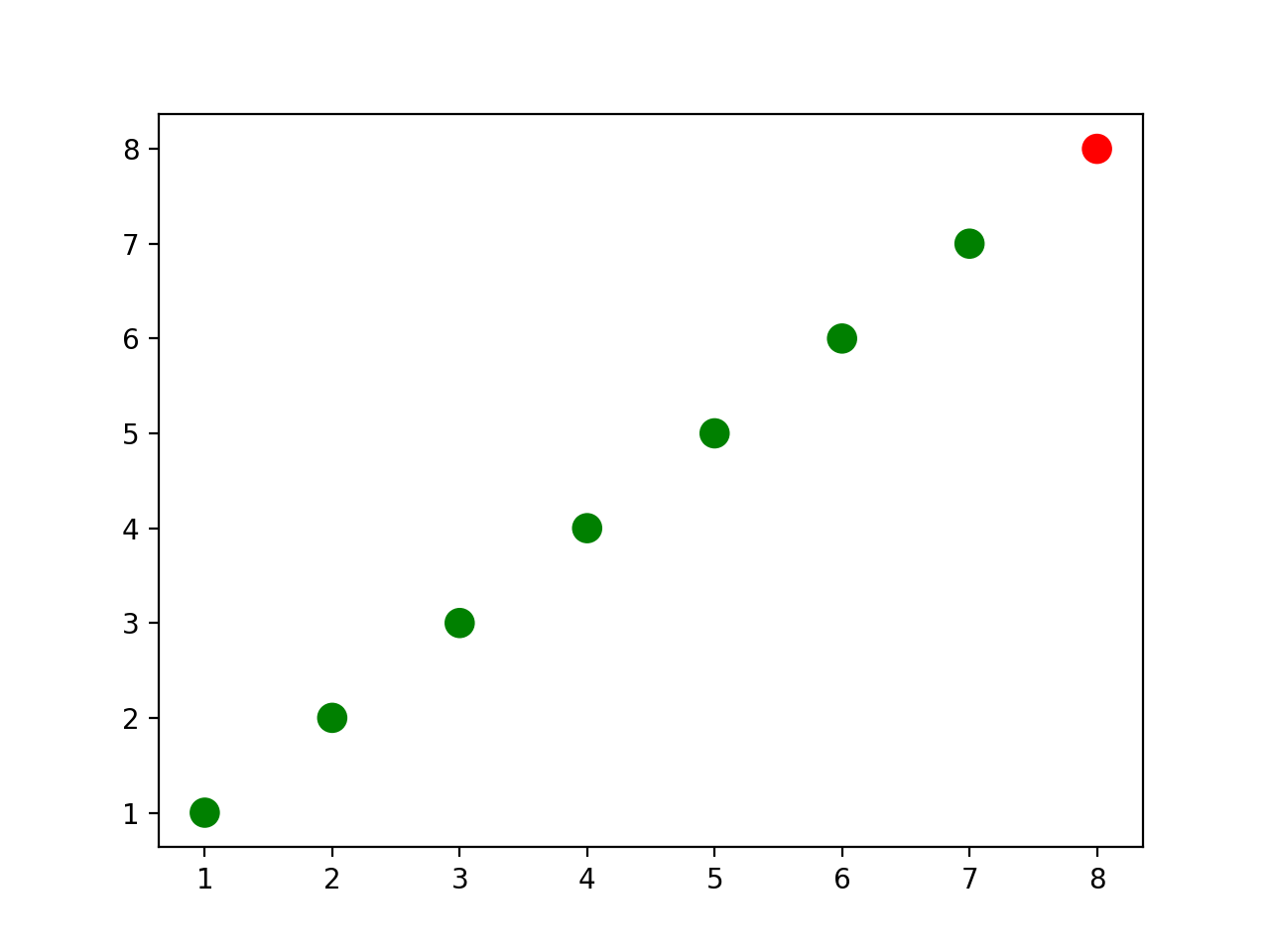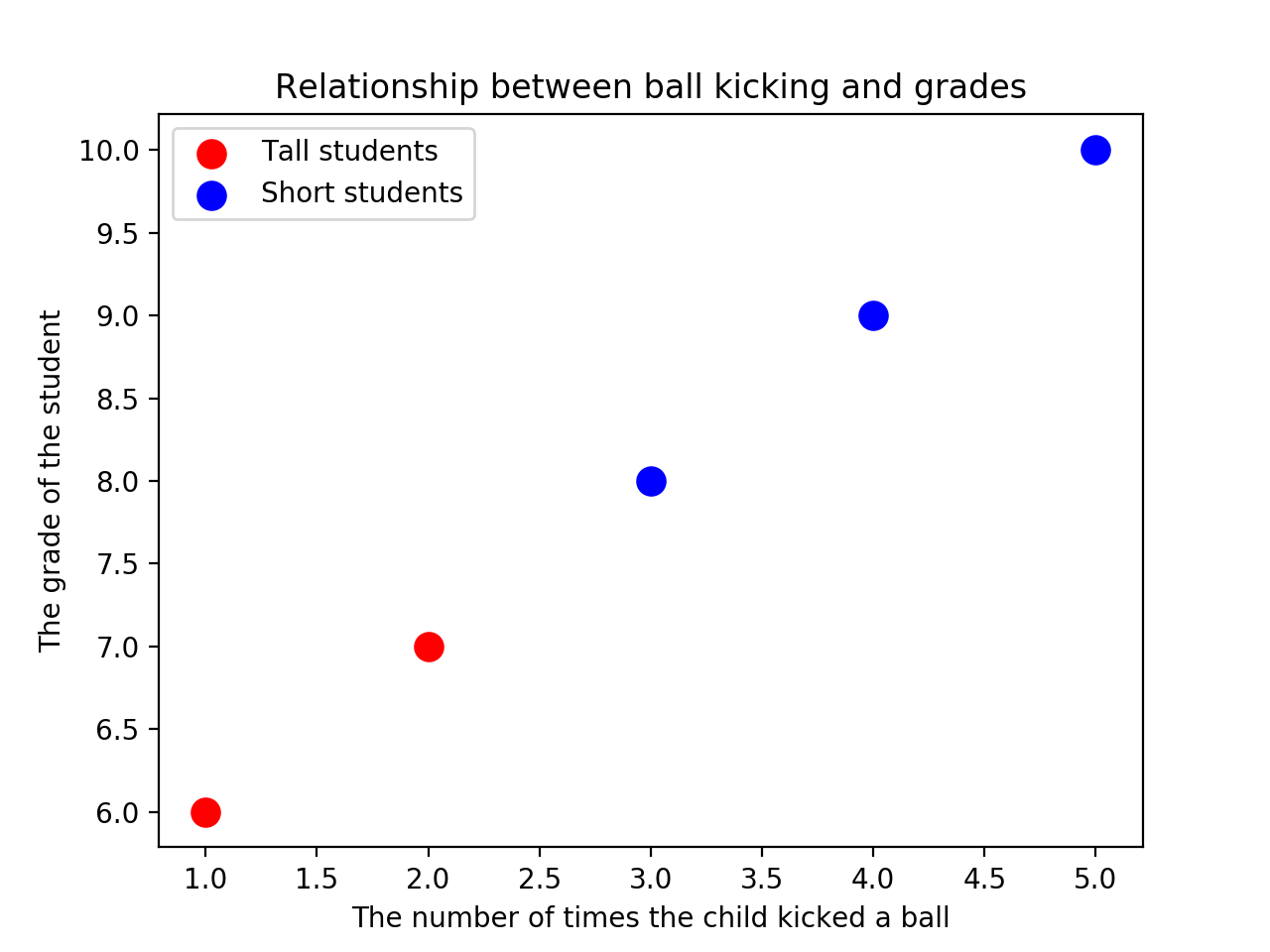# Scatterplots

Instructions below will leverage the Matplotlib “Scripting” layer that was described in Matplotlib Architecture. As written in those notes:

1. Pyplot (plt) retrieves the current figure with .gcf() and the current figure with .gca().
2. Pyplot “mirrors” the API of the axes object, so we can call .plot() function against the pyplot module (using plt.plot(), but this is calling ax.plot() underneath.
3. Functions in matplotlib generally end with an open set of keyword arguments, meaning there are a lot of different properties that can be controlled (Axes.plot(self, *args, scalex=True, scaley=True, data=None, **kwargs)).

### Scatterplots in Matplotlib

The scatter function is similar to plt.plot(x, y, '.'), but the underlying child objects in the axes are not Line2D.

%matplotlib notebook

import matplotlib.pyplot as plt
import numpy as np

x = np.array([1,2,3,4,5,6,7,8])
y = x

plt.figure()
plt.scatter(x, y);

<IPython.core.display.Javascript object>#### Set some colors and increase size.

colors = ['green']*(len(x)-1)
colors.append('red')

plt.figure()

plt.scatter(x, y, s=100, c=colors);

<IPython.core.display.Javascript object>#### Change points and colors, label axes, add legend.

Rather than two lists, use a single list of pairwise tuples, created using the builtin zip.

zip takes a number of iterables and creates tuples out of them, matching based on index. zip has lazy evaluation, so use a list typecase to view the results.

zip_generator = zip([1,2,3,4,5], [6,7,8,9,10])
print(zip_generator)
print(list(zip_generator))

<zip object at 0x11d37de08>
[(1, 6), (2, 7), (3, 8), (4, 9), (5, 10)]


The * unpacks the collection into positional arguments.

zip_generator = zip([1,2,3,4,5], [6,7,8,9,10])
print(*zip_generator)

(1, 6) (2, 7) (3, 8) (4, 9) (5, 10)

zip_generator = zip([1,2,3,4,5], [6,7,8,9,10])
x, y = zip(*zip_generator)
print(x)
print(y)

(1, 2, 3, 4, 5)
(6, 7, 8, 9, 10)

plt.figure()
plt.scatter(x[:2], y[:2], s=100, c='red', label='Tall students')
plt.scatter(x[2:], y[2:], s=100, c='blue', label='Short students')
plt.xlabel('The number of times the child kicked a ball')
plt.title('Relationship between ball kicking and grades')
plt.legend(loc=4, frameon=False, title='Legend');

<IPython.core.display.Javascript object>#### Unpack the Artists in this visual

(plt
.gca()
.get_children())

[<matplotlib.collections.PathCollection at 0x11d3bf128>,
<matplotlib.collections.PathCollection at 0x11d3bf550>,
<matplotlib.spines.Spine at 0x11201c9b0>,
<matplotlib.spines.Spine at 0x11201c438>,
<matplotlib.spines.Spine at 0x11d391400>,
<matplotlib.spines.Spine at 0x11d391470>,
<matplotlib.axis.XAxis at 0x11201c7f0>,
<matplotlib.axis.YAxis at 0x11d391828>,
Text(0.5, 1.0, 'Relationship between ball kicking and grades'),
Text(0.0, 1.0, ''),
Text(1.0, 1.0, ''),
<matplotlib.legend.Legend at 0x11d3a2a90>,
<matplotlib.patches.Rectangle at 0x11d3a2ac8>]


The legened is the second to last item in this list.

legend = (plt
.gca()
.get_children()[-2])


The artists have child objects, as well.

(legend
.get_children()
.get_children()
.get_children()
.get_children())

[<matplotlib.offsetbox.HPacker at 0x11d3ce0b8>,
<matplotlib.offsetbox.HPacker at 0x11d3ce0f0>]


The following function prints all the artists a given artist is made of.

# import the artist class from matplotlib
from matplotlib.artist import Artist

def rec_gc(art, depth=0):
if isinstance(art, Artist):
# increase the depth for pretty printing
print(" " * depth + str(art))
for child in art.get_children():
rec_gc(child, depth+2)


Call it on the legend.

rec_gc(plt.legend())

Legend
<matplotlib.offsetbox.VPacker object at 0x11d3cee48>
<matplotlib.offsetbox.TextArea object at 0x11d3cecf8>
Text(0, 0, '')
<matplotlib.offsetbox.HPacker object at 0x11d3cecc0>
<matplotlib.offsetbox.VPacker object at 0x11d3cec18>
<matplotlib.offsetbox.HPacker object at 0x11d3cec50>
<matplotlib.offsetbox.DrawingArea object at 0x11d3ce908>
<matplotlib.collections.PathCollection object at 0x11d3bffd0>
<matplotlib.offsetbox.TextArea object at 0x11d3ce390>
Text(0, 0, 'Tall students')
<matplotlib.offsetbox.HPacker object at 0x11d3cec88>
<matplotlib.offsetbox.DrawingArea object at 0x11d3ceac8>
<matplotlib.collections.PathCollection object at 0x11d3ce9b0>
<matplotlib.offsetbox.TextArea object at 0x11d3cea20>
Text(0, 0, 'Short students')
FancyBboxPatch((0, 0), width=1, height=1)


So, a legend artist is made of offset boxes for drawing, text areas, and path collections.

Calls to the matplot lib scripting interface create figures, subplots, and axes. These artists are loaded into axes objects, which the back-end renders to the screen or to a file.